# Author Archive | Michael Parker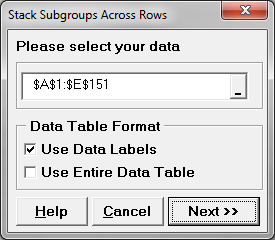## Attribute MSA with SigmaXL

Use SigmaXL to Implement an Attribute MSA Data File: “Attribute MSA” tab in “Sample Data.xlsx” (an example in the AIAG MSA Reference Manual, 3rd Edition). Step 1: Reorganize the original data into four new columns (i.e., Appraiser, Assessed Result, Part, and Reference). Select the entire range of the original data (“Part”, “Reference”, “Appraiser A”, “Appraiser […]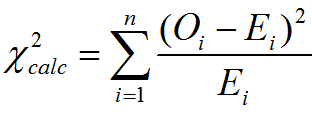## Chi Square Tests with SigmaXL

Chi Square (Contingency Tables) We have looked at hypothesis tests to analyze the proportion of one population vs. a specified value, and the proportions of two populations, but what do we do if we want to analyze more than two populations? A chi-square test is a hypothesis test in which the sampling distribution of the […]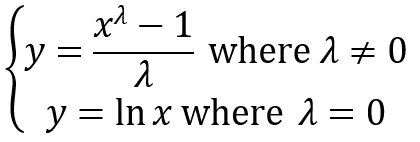## Box Cox Transformation with SigmaXL

Box Cox Transformation Box Cox Transformation Formula The formula of the Box Cox transformation is: Where: Use SigmaXL to Perform a Box-Cox Transformation SigmaXL provides the best Box-Cox transformation with an optimal λ that minimizes the model SSE (sum of squared error). Here is an example of how we transform the non-normally distributed response to […]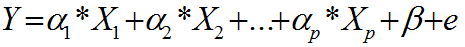## Multiple Linear Regression

What is Multiple Linear Regression? Multiple linear regression is a statistical technique to model the relationship between one dependent variable and two or more independent variables by fitting the data set into a linear equation. The difference between simple linear regression and multiple linear regression: Multiple Linear Regression Equation Where: Both dependent and independent variables […]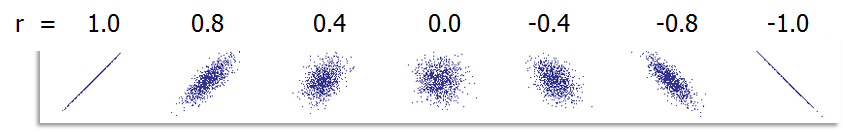## Correlation Coefficient with SigmaXL

Pearson’s Correlation Coefficient Pearson’s correlation coefficient is also called Pearson’s r or coefficient of correlation and Pearson’s product moment correlation coefficient (r), where r is a statistic measuring the linear relationship between two variables. What is Correlation? Correlation is a statistical technique that describes whether and how strongly two or more variables are related. Correlation […]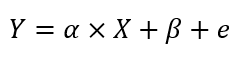## Simple Linear Regression

What is Simple Linear Regression? Simple linear regression is a statistical technique to fit a straight line through the data points. It models the quantitative relationship between two variables. It is simple because only one predictor variable is involved. It describes how one variable changes according to the change of another variable. Both variables need […]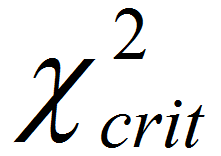## Median Test with SigmaXL

What is Mood’s Median Test? Mood’s median test is a statistical test to compare the medians of two or more populations. The symbol k is the number of groups of our interest and is equal to or greater than two. Mood’s median is an alternative to Kruskal–Wallis. For the data with outliers, Mood’s median test […]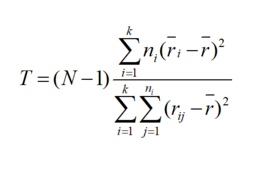## Kruskal Wallis with SigmaXL

Kruskal–Wallis One-Way Analysis of Variance The Kruskal Wallis one-way analysis of variance is a statistical hypothesis test to compare the medians among more than two groups. It is an extension of Mann–Whitney test. While the Mann–Whitney test allows us to compare the samples of two populations, the Kruskal–Wallis test allows us to compare the samples […]